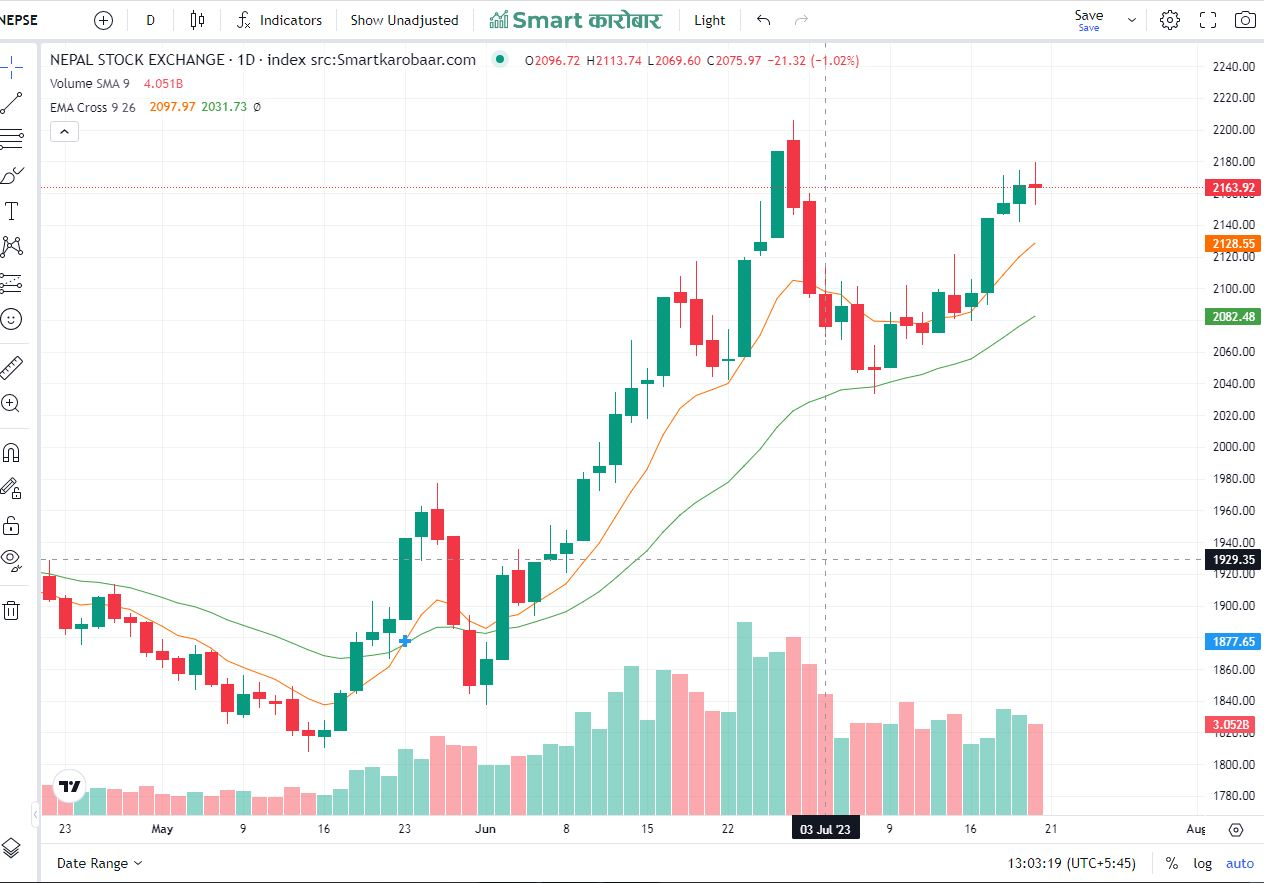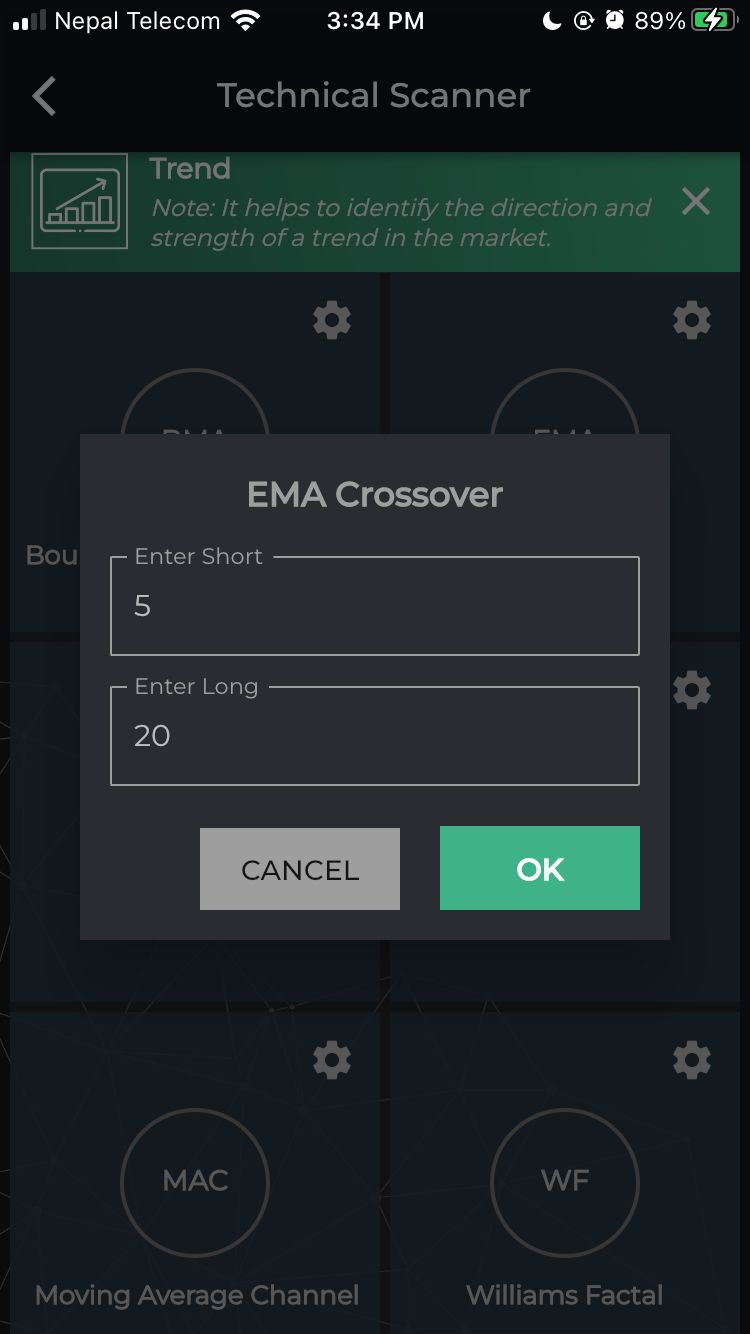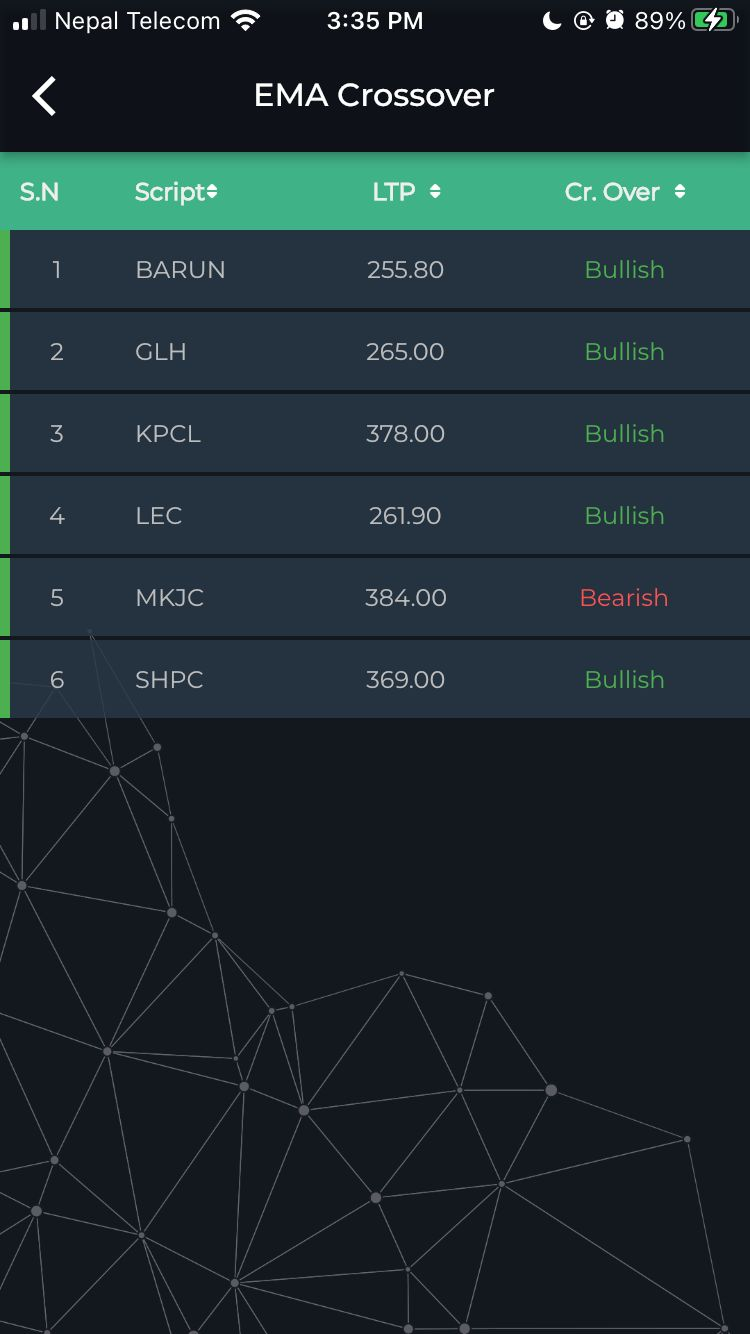# Understanding the EMA Crossover Strategy

The Exponential Moving Average (EMA) is a type of moving average that gives more weight to recent price data, making it more responsive to market changes. The EMA crossover strategy involves plotting two EMAs on a price chart – one with a shorter period and another with a longer period. The most common EMA crossover used by traders is the 12-day EMA and the 26-day EMA.

When the shorter-term EMA crosses above the longer-term EMA, it generates a bullish signal, indicating a potential uptrend in the market. Conversely, when the shorter-term EMA crosses below the longer-term EMA, it generates a bearish signal, indicating a potential downtrend in the market. Traders use these crossovers to time their entry and exit points for trades.## To calculate EMA crossovers manually:

Step 1: Gather Historical Price Data

Collect historical price data for the financial instrument you want to analyze. The data typically includes the daily closing prices over the desired time period.

Step 2: Choose EMA Periods

Determine the EMA periods you want to use. The most common EMA crossover used in trading is the 12-day EMA and the 26-day EMA.

Step 3: Calculate the EMAs

Calculate the EMA values for both the chosen periods (e.g., 12-day and 26-day EMAs). To calculate the EMA, use the formula provided in the previous response:

EMA = (Closing Price - Previous EMA) * Multiplier + Previous EMA

Where the multiplier is calculated as follows:

For the 12-day EMA: Multiplier = 2 / (12 + 1) = 0.1538

For the 26-day EMA: Multiplier = 2 / (26 + 1) = 0.0741

Calculate the first EMA values using the respective period's simple moving average as the starting point.

Step 4: Identify Crossovers

An EMA crossover occurs when the 12-day EMA line crosses above or below the 26-day EMA line. When the 12-day EMA crosses above the 26-day EMA, it generates a bullish signal, indicating a potential uptrend. Conversely, when the 12-day EMA crosses below the 26-day EMA, it generates a bearish signal, indicating a potential downtrend.

Step 5: Analyze Crossover Signals

Analyze the crossover signals to make trading decisions. Some traders may consider a bullish crossover as a signal to enter a long (buy) position, while a bearish crossover may signal an opportunity to enter a short (sell) position.

You can scan EMA crossover using smartkarobaar app :